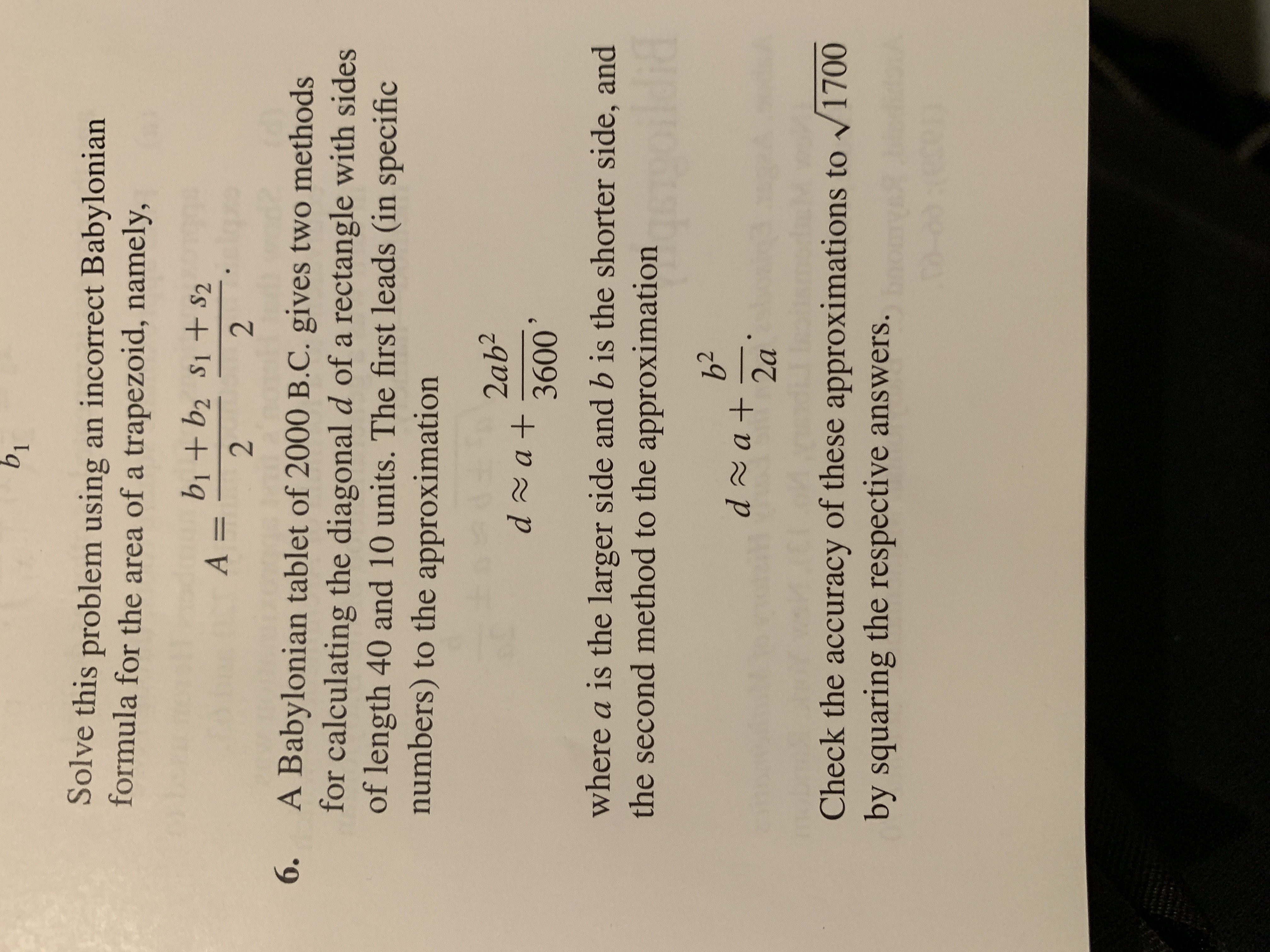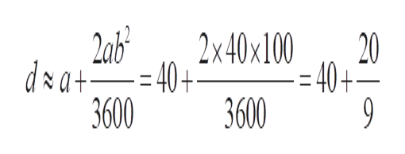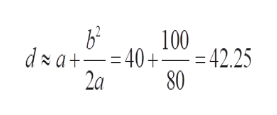Solve this problem using an incorrect Babylonianformula for the area of a trapezoid, namely,b1 + b2 S1 + S2A =22A Babylonian tablet of 2000 B.C. gives two methodsfor calculating the diagonal d of a rectangle with sidesof length 40 and 10 units. The first leads (in specificnumbers) to the approximation2ab2d a+3600where a is the larger side and b is the shorter side, andthe second method to the approximationd at2aCheck the accuracy of these approximations to V1700by squaring the respective answers.

Questionhelp_outlineImage TranscriptioncloseSolve this problem using an incorrect Babylonian formula for the area of a trapezoid, namely, b1 + b2 S1 + S2 A = 2 2 A Babylonian tablet of 2000 B.C. gives two methods for calculating the diagonal d of a rectangle with sides of length 40 and 10 units. The first leads (in specific numbers) to the approximation 2ab2 d a+ 3600 where a is the larger side and b is the shorter side, and the second method to the approximation d at 2a Check the accuracy of these approximations to V1700 by squaring the respective answers. fullscreen
Step 1

To analyze the accuracy of the Babylonian tablet

Step 2

1st method: d is approximately 42.22222..., whose square is 1782.716help_outlineImage Transcriptioncloseab3 2x40x100 20 dxa 40+ - -40+ -40+= 3600 3600 fullscreen
Step 3

In the second method, d is estimated by ...help_outlineImage Transcriptioncloseb2 100 d a+40+ 42.25 2a 80 fullscreen

Want to see the full answer?

See Solution

Want to see this answer and more?

Our solutions are written by experts, many with advanced degrees, and available 24/7

See Solution
Tagged in

Math